Next: Problems Up: construct Previous: Simple Algebraic Concepts

# Three Impossible Constructions

The three classical construction problems of antiquity are known as squaring the circle'', trisecting an angle'', and doubling a cube''. Here is a short description of each of these three problems:

• Squaring the Circle. Given a circle, construct a square that has exactly the same area as the circle.
• Trisecting an Angle. Given an angle, construct an angle whose measure is exactly 1/3 the measure of the original angle.
• Doubling a Cube. Given the length of the side of a (three-dimensional) cube, constuct a length so that a cube with an edge of this length will have exactly double the area of the original cube.

It is beyond the scope of this paper to prove that these three problems are impossible to solve, but given the ideas that were presented in Section 5, we can at least indicate how the formal proofs work.

The easiest to show (to be impossible) is the problem of doubling the cube. Clearly if the volume of the required cube is double the volume of the original cube, if the length of the side of the original cube is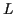, the desired length is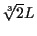.

In other words, from an algebraic point of view, we can construct the cube root of 2, or equivalently, we can solve the following equation: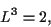which is irreducible over the rationals, and is not going to be in any quadratic extension of the rationals.

The problem of trisecting an angle is similar, but what is usually done is to show that there is a particular angle that cannot be trisected, and that angle is typically chosen to be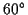. If we can't trisect this particular one, then we know that not all angles can be trisected. (Remember that a solution to the problem requires a general method that will work for any angle. Certain angles, such as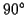angles, can obviously be trisected, since we can construct a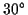angle from scratch. Also, we can construct aangle from scratch, so if this one cannot be trisected, the general problem of trisection is clearly unsolvable.)

If we can trisect aangle, that is equivalent to constructing a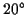angle from scratch, which is equivalent to constructing the cosine of.

Now the cosine ofis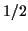, so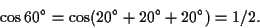Using the standard formulas for the sine and cosine of sums of angles, this equation above can be converted to: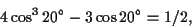or if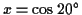, to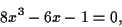which is also an irreducible cubic equation that cannot possibly have a solution in any quadratic extension of the rationals.

Much more difficult is to show that a circle cannot be squared. If the original circle has radius 1, its area will be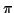, so squaring a circle is equivalent to the construction of a length equal to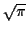. It turns out (but is not easy to prove) thatis transcendental--it is not the solution to any polynomial equation, so it is surely not the solution to any combination of quadratic polynomials.Next: Problems Up: construct Previous: Simple Algebraic Concepts
Zvezdelina Stankova-Frenkel 2000-11-13